# Machine Learning

### K-Means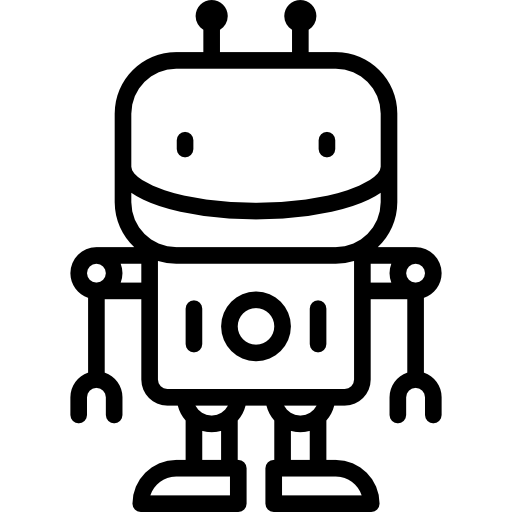## Centroid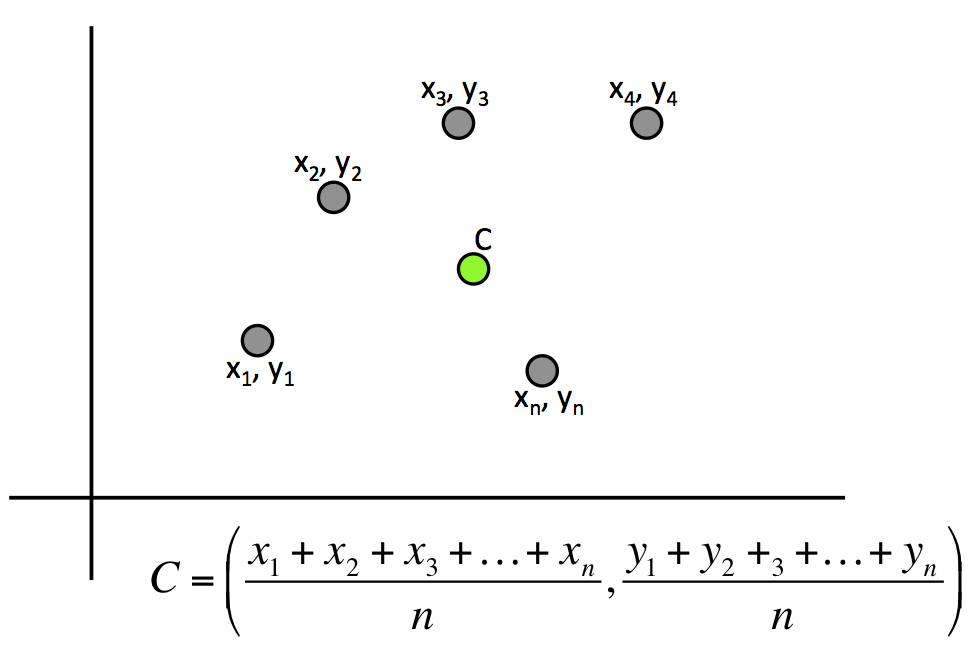## Active Learning

Calculate centroid ...

## K-Means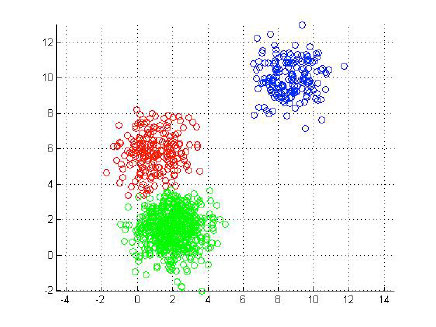## K-Means Algorithm

1. Place K points into the space represented by the objects. These points represent initial group of centroids.

2. Assign each object to the group that has the closest centroid.

3. When all objects have been assigned, recalculate the positions of the K centroids.

4. Repeat Steps 2 and 3 until the centroids no longer move.

## K-Means Algorithm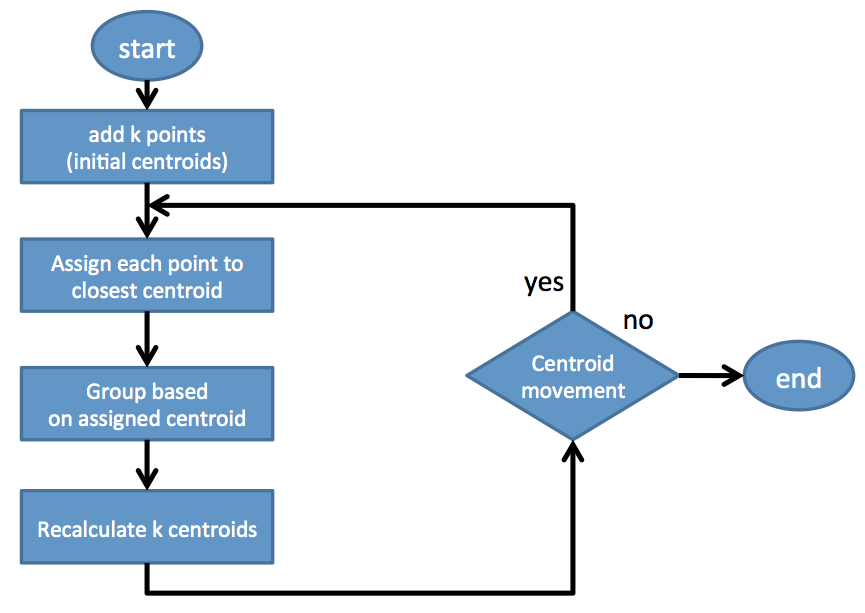## Visualization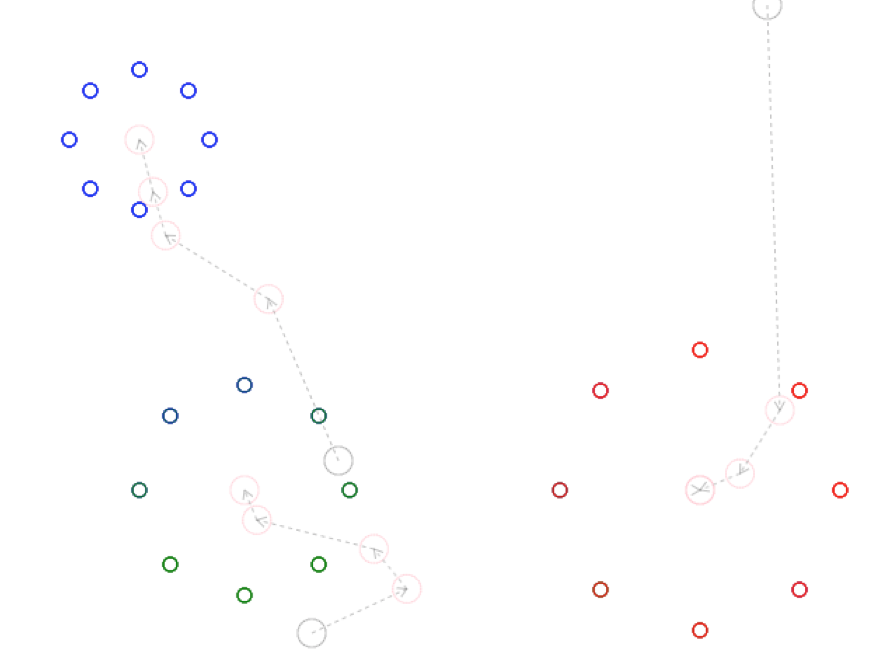## Active Learning

Write an implementation of K-Means …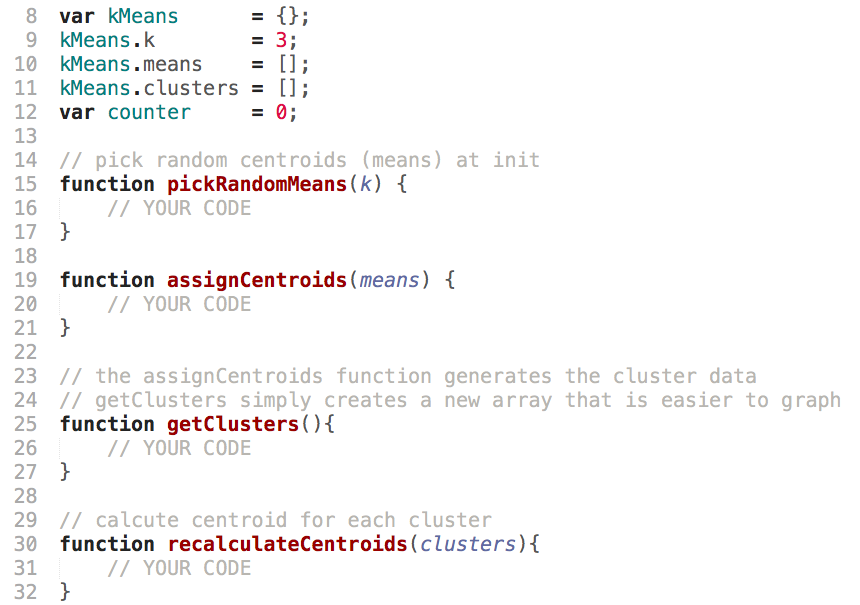## Distance Between Two Points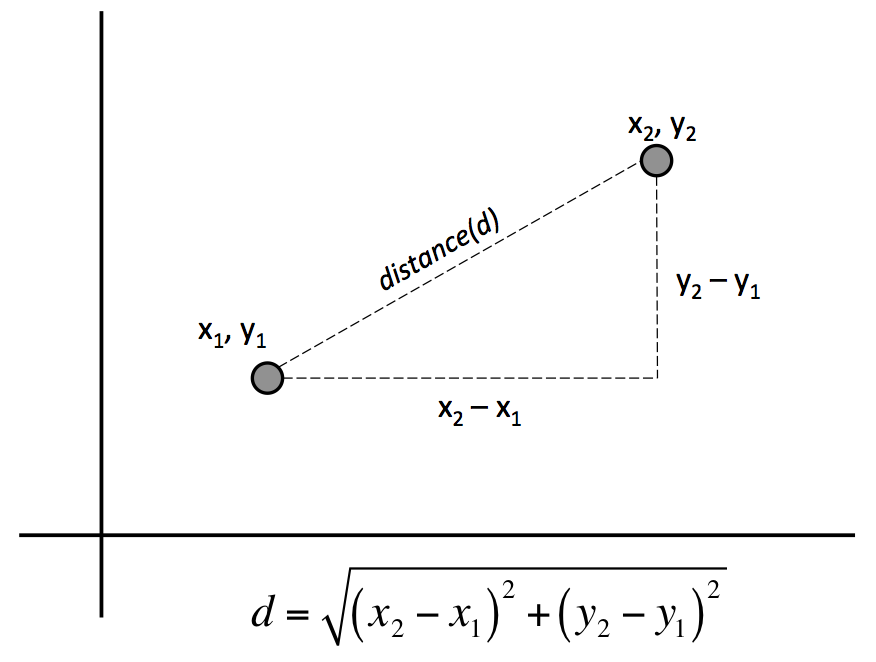## Centroid